Homework Help Question & Answers

# KIL : Enter 4 5 6 6. What is the electron configuration and outer shell orbital...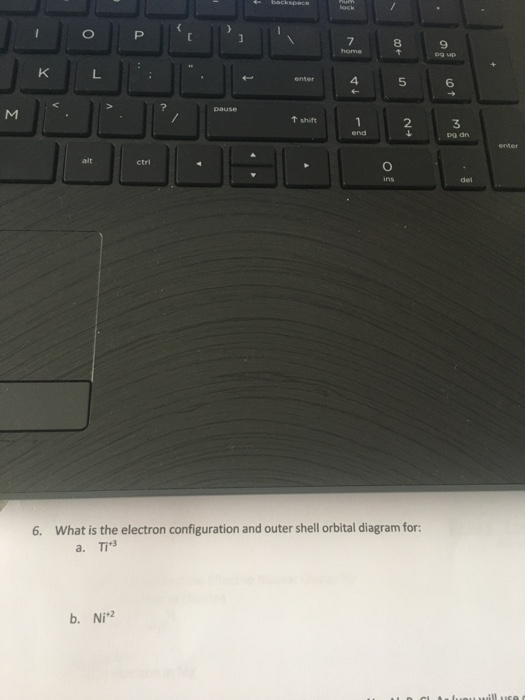KIL : Enter 4 5 6 6. What is the electron configuration and outer shell orbital diagram for: a. T1" b. Nj"

#### Homework Answers

Answer #1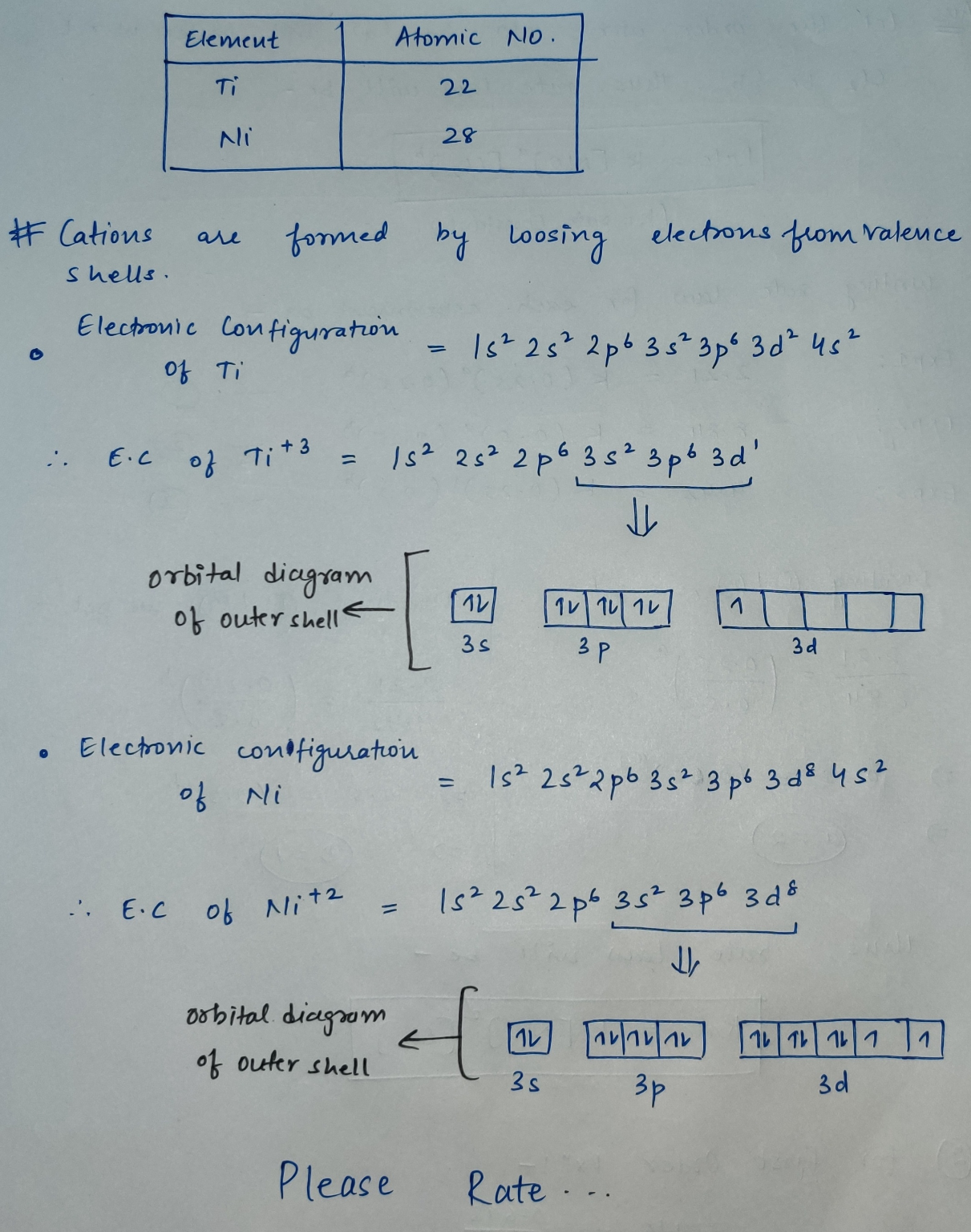Know the answer?
Your Answer:

#### Post as a guest

Your Name:

What's your source?

#### Earn Coin

Coins can be redeemed for fabulous gifts.

Not the answer you're looking for? Ask your own homework help question. Our experts will answer your question WITHIN MINUTES for Free.
Similar Homework Help Questions
• ### Orbital Diagrams Construct the orbital diagram of each atom or ion. What is the electron configuration...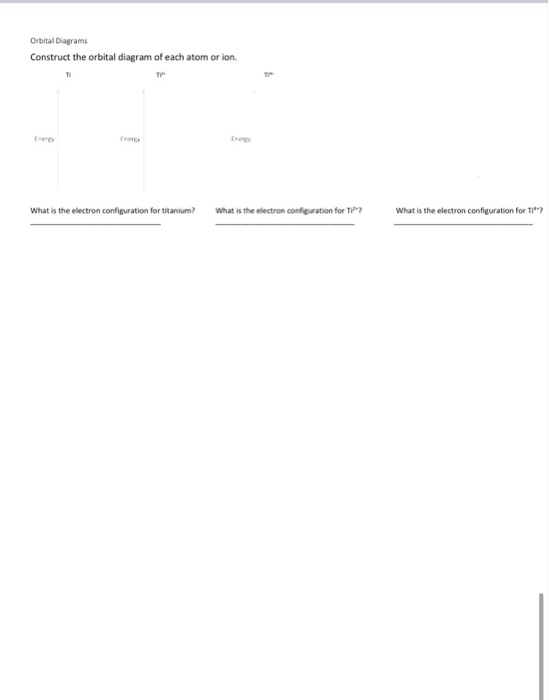Orbital Diagrams Construct the orbital diagram of each atom or ion. What is the electron configuration for titanium? What is the electron configuration for i?? What is the electron configuration for Time

• ### 4. (30 points) What is the Lewis symbol for carbon? Write the electron configuration and orbital...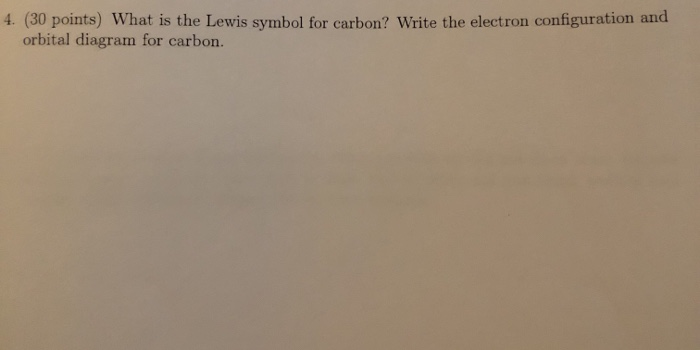4. (30 points) What is the Lewis symbol for carbon? Write the electron configuration and orbital diagram for carbon.

• ### (5 points) (a) Write the short-hand electron configuration of Vanadium. (b) Draw the orbital diagram of...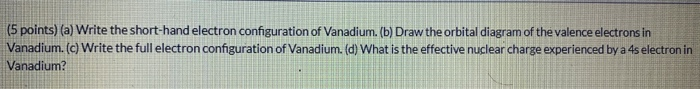(5 points) (a) Write the short-hand electron configuration of Vanadium. (b) Draw the orbital diagram of the valence electrons in Vanadium. (c) Write the full electron configuration of Vanadium. (d) What is the effective nuclear charge experienced by a 4s electron in Vanadium?

• ### Using the electron configuration scheme, answer all of the following: 1. write the orbital filling diagram...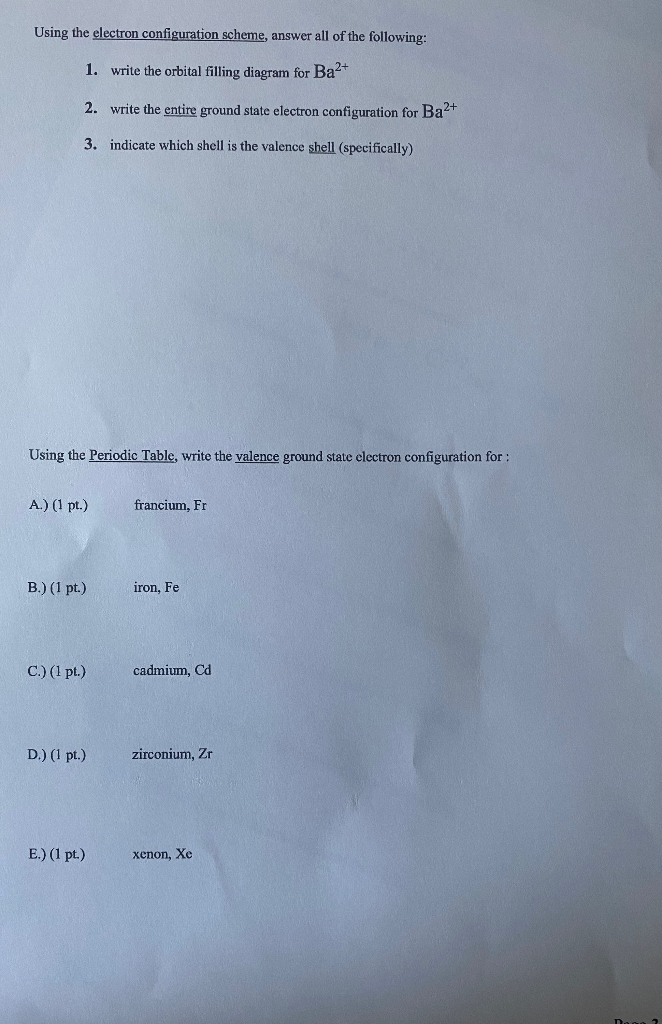Using the electron configuration scheme, answer all of the following: 1. write the orbital filling diagram for Ba2+ 2. write the entire ground state electron configuration for Ba2+ 3. indicate which shell is the valence shell (specifically) Using the Periodic Table, write the valence ground state electron configuration for : A.) (1 pt.) francium, Fr B.) (1 pt.) iron, Fe C.) (1 pt.) cadmium, ca D.) (1 pt.) zirconium, Zr E.) (1 pt.) xenon, Xe

• ### Electron Configuration and Orbital diagrams

Write electron configuration and orbital diagram forAu+?Thank you!

• ### 6.(18ps) (a) 6pts. What is the ground state electron configuration and orbital diagram of Nb(41)? Is...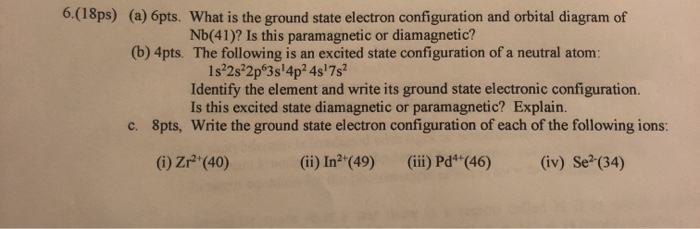6.(18ps) (a) 6pts. What is the ground state electron configuration and orbital diagram of Nb(41)? Is this paramagnetic or diamagnetic? (b) 4pts. The following is an excited state configuration of a neutral atom: 1s2s22p 3s!4p2 4s 7s Identify the element and write its ground state electronic configuration. Is this excited state diamagnetic or paramagnetic? Explain. 8pts, Write the ground state electron configuration of each of the following ions: c. (iv) Se (34) (ii) In2'(49) (ii) Pd*(46) (i) Zr (40)

• ### 6.(18ps) (a) 6pts. What is the ground state electron configuration and orbital diagram of Nb(41)? Is...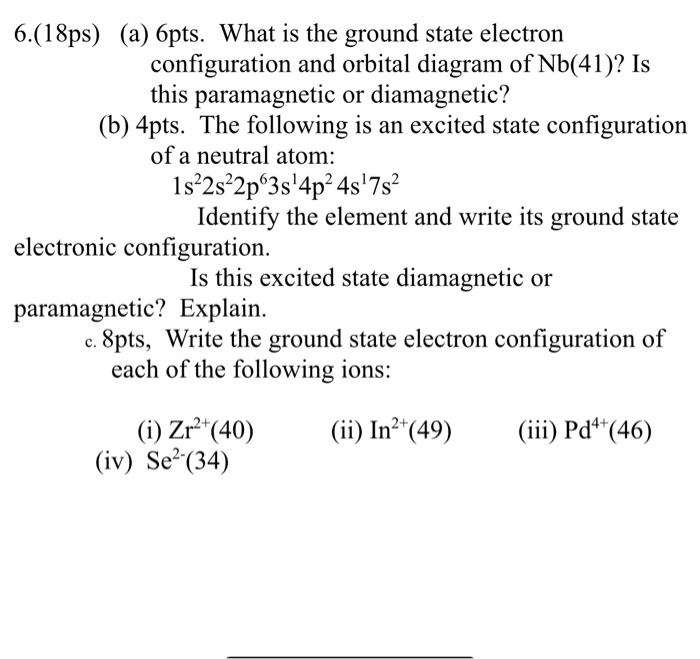6.(18ps) (a) 6pts. What is the ground state electron configuration and orbital diagram of Nb(41)? Is this paramagnetic or diamagnetic? (b) 4pts. The following is an excited state configuration of a neutral atom: 1s²2s²2pº3s'4p2 45'7s Identify the element and write its ground state electronic configuration. Is this excited state diamagnetic or paramagnetic? Explain. c. 8pts, Write the ground state electron configuration of each of the following ions: (ii) In2+(49) (iii) Pd4+(46) (i) Zr2+(40) (iv) Se?(34)

• ### 6.(18ps) (a) 6pts. What is the ground state electron configuration and orbital diagram of Nb(41)? Is...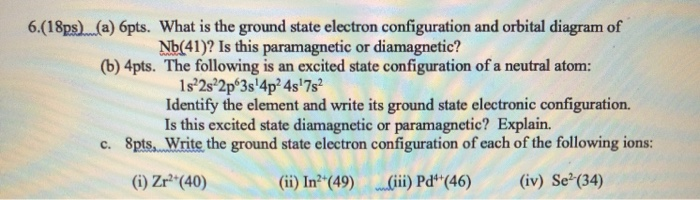6.(18ps) (a) 6pts. What is the ground state electron configuration and orbital diagram of Nb(41)? Is this paramagnetic or diamagnetic? (b) 4pts. The following is an excited state configuration of a neutral atom: 1s 2s 2p 3s'4p24s'7s2 Identify the element and write its ground state electronic configuration. Is this excited state diamagnetic or paramagnetic? Explain. c. Spts. Write the ground state electron configuration of each of the following ions: (1) Zr?*(40) (ii) In?*(49) Ciii) Pd**(46) (iv) Se?(34)

• ### Provide the orbital diagram, electron configuration, condensed electron configuration, and the number of unpaired electrons for...

Provide the orbital diagram, electron configuration, condensed electron configuration, and the number of unpaired electrons for ground state (a) oxygen, (b) titanium and (c) chromium. Discussion: How would you use the periodic table above to help fill the orbitals of many-electron atoms? Which atoms on the first row of the transition metals have anomalous electron configurations?

• ### Write the i) full electron configuration ii) the orbital filling diagram and iii) the condensed electron...

Write the i) full electron configuration ii) the orbital filling diagram and iii) the condensed electron configuration for: a) Copper b) Bromine